Email us to get an instant 20% discount on highly effective K-12 Math & English kwizNET Programs!

#### Online Quiz (WorksheetABCD)

Questions Per Quiz = 2 4 6 8 10

### High School Mathematics8.6 Domain and Range of a Relation using Diagrams

 Directions: Choose the correct answer for the following. Also write at least ten examples of your own.
 Q 1: Using the below diagram find the domain.{x,y,z}{a,b,c} Q 2: Using the below diagram find the range.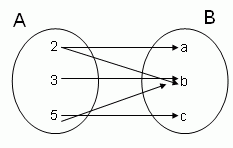{2,3,5}{1,2,3} Q 3: Using the below diagram find the domain.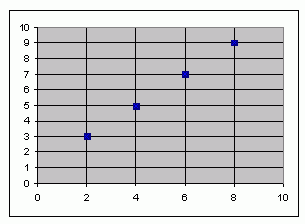{3,5,7,9}{2,4,6,8} Q 4: Using the below diagram find the range.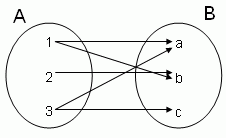{1,2,3}{a,b,c} Q 5: Using the below diagram find the domain.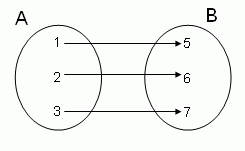{1,2,3}{5,7,9} Q 6: Using the below diagram find the domain.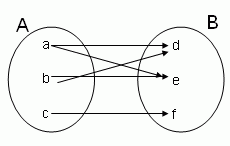{d,e,f}{a,b,c} Question 7: This question is available to subscribers only! Question 8: This question is available to subscribers only!

#### Subscription to kwizNET Learning System offers the following benefits:

• Unrestricted access to grade appropriate lessons, quizzes, & printable worksheets
• Instant scoring of online quizzes
• Progress tracking and award certificates to keep your student motivated
• Unlimited practice with auto-generated 'WIZ MATH' quizzes